# Precalculus : Graphs of Polynomial Functions

## Example Questions

2 Next →

### Example Question #3 : Write The Equation Of A Polynomial Function Based On Its Graph

Write the quadratic function for the graph: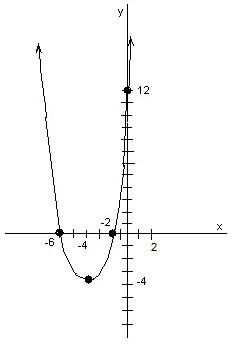Possible Answers: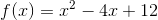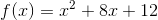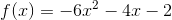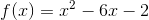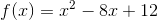Correct answer:Explanation:

Method 1:

The x-intercepts are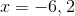.  These values would be obtained if the original quadratic were factored, or reverse-FOILed and the factors were set equal to zero.

For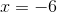,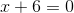.  For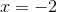,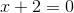.  These equations determine the resulting factors and the resulting function;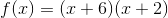.

Multiplying the factors and simplifying,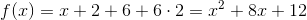.

Answer:.

Method 2:

Use the form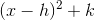, where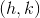is the vertex.is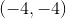, so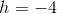,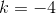.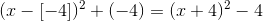Answer: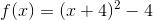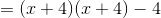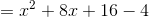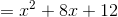### Example Question #4 : Write The Equation Of A Polynomial Function Based On Its Graph

Write the equation for the polynomial in this graph: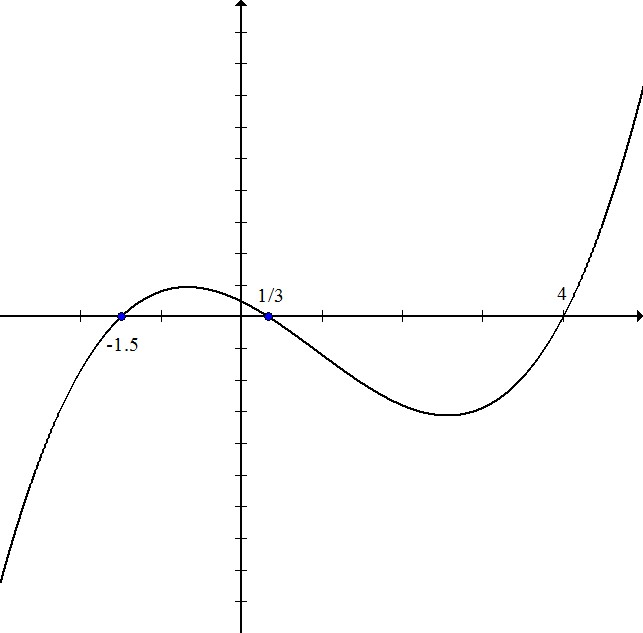Possible Answers: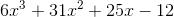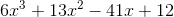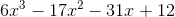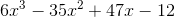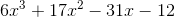Correct answer:Explanation:

The zeros for this polynomial are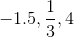.

This means that the factors are equal to zero when these values are plugged in for x.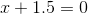multiply both sides by 2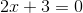so one factor is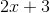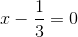multiply both sides by 3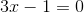so one factor is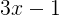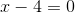so one factor is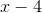Multiply these three factors: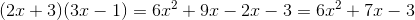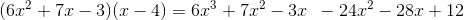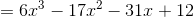### Example Question #5 : Write The Equation Of A Polynomial Function Based On Its Graph

Write the equation for the polynomial shown in this graph: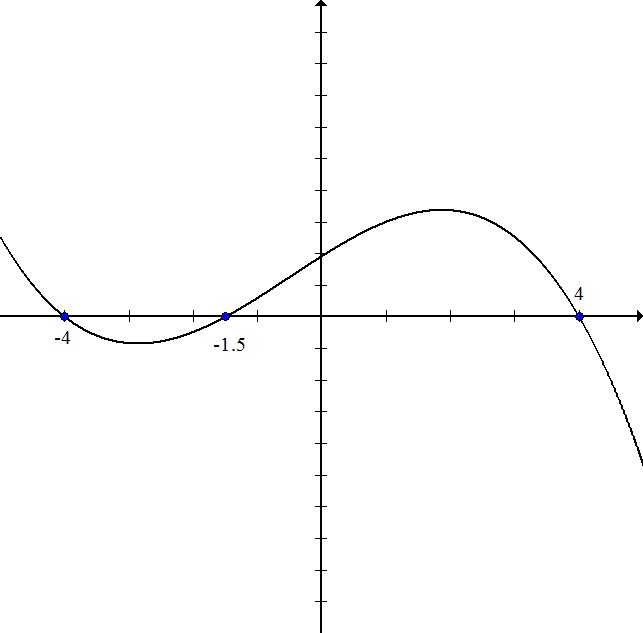Possible Answers: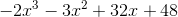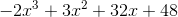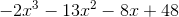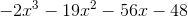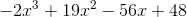Correct answer:Explanation:

The zeros of this polynomial are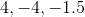. This means that the factors equal zero when these values are plugged in.

One factor isOne factor is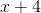The third factor is equivalent to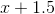. Set equal to 0 and multiply by 2: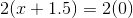Multiply these three factors: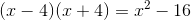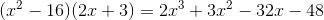The graph is negative since it goes down then up then down, so we have to switch all of the signs: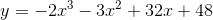### Example Question #6 : Write The Equation Of A Polynomial Function Based On Its Graph

Write the equation for the polynomial in the graph: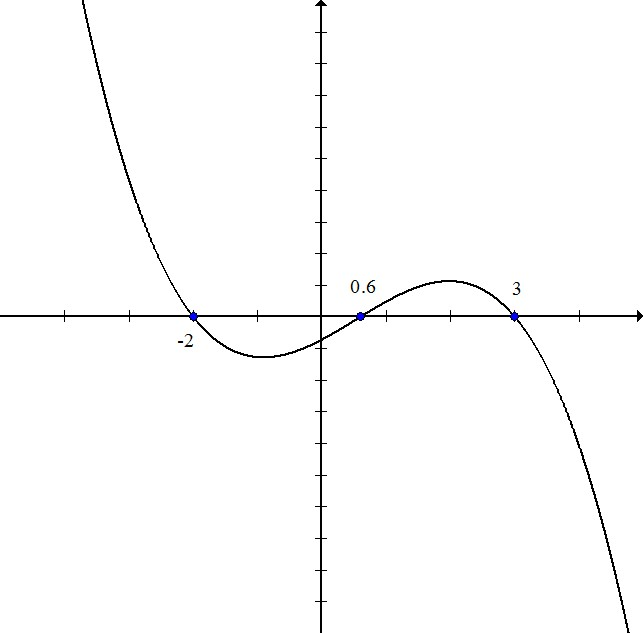Possible Answers: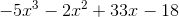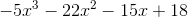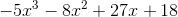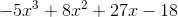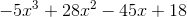Correct answer:Explanation:

The zeros of the polynomial are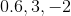. That means that the factors equal zero when these values are plugged in.

The first factor is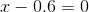or equivalently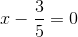multiply both sides by 5: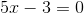The second and third factors are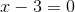andMultiply: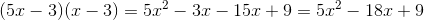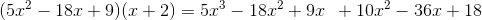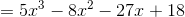Because the graph goes down-up-down instead of the standard up-down-up, the graph is negative, so change all of the signs:### Example Question #7 : Write The Equation Of A Polynomial Function Based On Its Graph

Write the equation for the polynomial in this graph: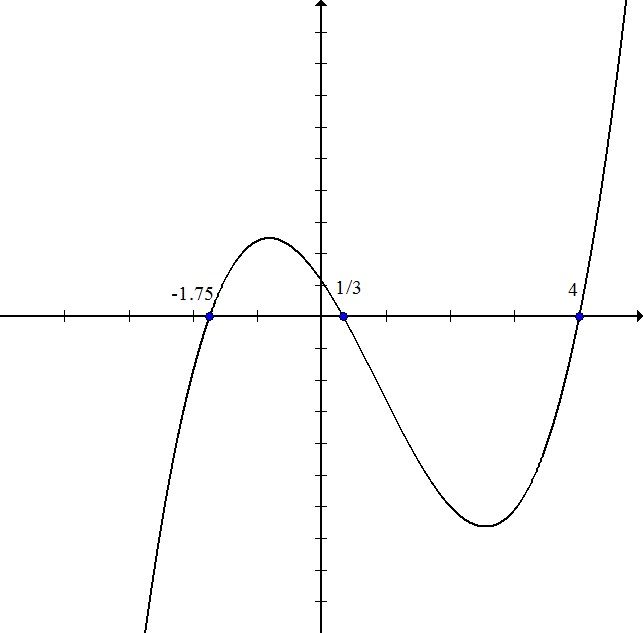Possible Answers: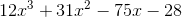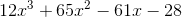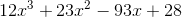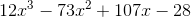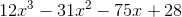Correct answer:Explanation:

The zeros for this polynomial are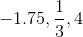. That means that the factors are equal to zero when these values are plugged in.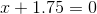or equivalently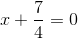multiply both sides by 4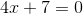the first factor is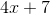multiply both sides by 3the second factor is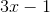the third factor isMultiply the three factors: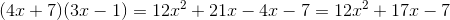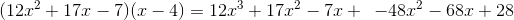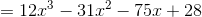2 Next →

### All Precalculus Resources